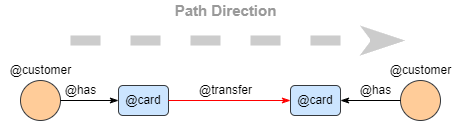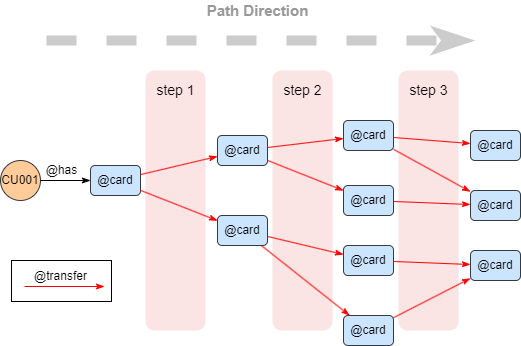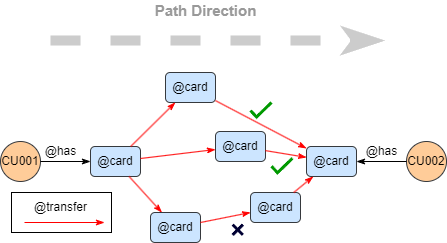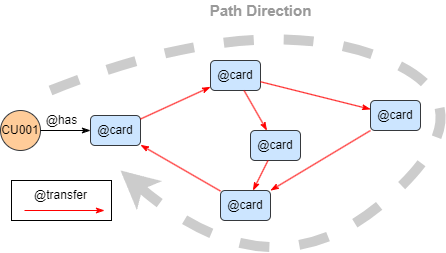# Change Nickname

Current Nickname:

Certifications

Certificate Issued at Valid until Serial No. File
Serial No. Valid until

Not having one? Apply now! >>>

Invoice

ProductName CreateTime ID Price File
ProductName CreateTime ID Price File
v4.0
Search
中文EN
v4.0

# Path Template

Path template query is a very flexible way of path query using path composed by basic templates.

Syntax:

• Command: None
• Parameter: None
• Statement Format: Path composed by basic templates
• Statement Alias: Custom alias supported, structure type is PATH
Parameter Type Specification Description Structure Type of Custom Alias
`no_circle()` / / To dismiss the paths with circles; see chapter Basic Concept for the definition of circle under Terminologies Not supported
`limit()` Int -1 or >=0 Number of results to return, -1 means to return all results Not supported

Path template query supports the prefix `optional`. With this prefix, a pseudo path "0-0-0" will be returned if there is no result found, and the value of the alias reference of any node or edge in the pseudo path is 0.

## 1 step

Example: as the path structure shown below, find transactions from a customer's card to another customer's card, return 10 paths and their properties``````n({@customer}).re({@has}).n({@card})
.re({@transfer}).n({@card})
.le({@has}).n({@customer}) as p
limit 10
return p{*}
``````

## N steps

Example: as the path structure shown below, find 3-step transaction paths from Customer CU001's card, return 10 paths and their properties``````n({_id == "CU001"}).re({@has}).n({@card})
.re({@transfer}).n({@card}) as p
limit 10
return p{*}
``````

## 1~N steps

Example: find 1 to 3 steps' transaction paths from Customer CU001's card, return 10 paths and their properties

``````n({_id == "CU001"}).re({@has}).n({@card})
.re({@transfer})[:3].n({@card})
.le({@has}).n({@customer}) as p
limit 10
return p{*}
``````

## M~N steps

Example: find 2 to 3 steps' transaction paths from Customer CU001's card, return 10 paths and their properties

``````n({_id == "CU001"}).re({@has}).n({@card})
.re({@transfer})[2:3].n({@card})
.le({@has}).n({@customer}) as p
limit 10
return p{*}
``````

## Non-weighted Shortest Path

Example: as the path structure shown below, find the shortest transaction paths from Customer CU001's card to Customer CU002's card wthin 3 steps, return 10 paths and their properties.``````n({_id == "CU001"}).re({@has}).n({@card} as n1)
n({_id == "CU002"}).re({@has}).n({@card} as n2)
with n1, n2
n(n1).re({@transfer})[*:3].n(n2) as p
limit 10
return p{*}
``````

Analysis: in this example, two path templates are first applied to find Customer CU001 and Customer CU002's cards respectively, then one card is used as `scr` and another as `dest` in a third transaction path template to find the shortest path between them.

## Circle

Example: as the path structure shown below, find the circular single-direction transaction paths from Customer CU001's card within 4 steps, return 10 paths and their properties``````n({_id == "CU001"}).re({@has}).n({@card} as n)
.re({@transfer})[:4].n(n) as p
limit 10
return p{*}
``````

## no_circle()

Example: find the non-circular single-direction transaction paths from Customer CU001's card within 4 steps, return 10 paths and their properties

``````n({_id == "CU001"}).re({@has}).n({@card})
.re({@transfer})[:4].n({@card})
.no_circle() as p
limit 10
return p{*}
``````

## limit

Example: find the transaction paths from Card CA001, CA002, and CAOO5 to Card CA016 within 3 steps, return 2 paths for each pair and their properties

``````find().nodes({_id in ["CA001", "CA002", "CA005"]}) as n
n(n).e()[:3].n({_id == "CA016"})
.limit(2) as p
return p{*}
``````

## OPTIONAL

Example: find the transaction paths from Card CA001, CA002, and CAOO5 to Card CA016 within 3 steps, return 2 paths for each pair and their properties; if no paths returned, return optional paths

``````find().nodes({_id in ["CA001", "CA002", "CA005"]}) as n
optional n(n).e()[:3].n({_id == "CA016"})
.limit(2) as p
return p{*}
``````

## 0~N steps  4.1

Example: find customers who viewed or purchased Product POO1, then find other customers 0 to 2 steps away from those customers, return all secondary customers' properties.

``````n({_id == "P001"}).e().n({@customer})
.e()[0:2].n({@customer}) as p
return p{*}
``````

## In-step Filter

Example: find the outbound @transfer paths from Card CA001 to Card CAOO2 wthin 4 steps, with each @transfer time greater than the previous one within the same path, return 10 paths and their properties.

``````n({_id == "CA001"}).re({@transfer.time > prev_e.time})[:4].n({_id == "CA002"}) as p
limit 10
return p{*}
``````